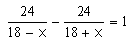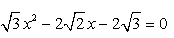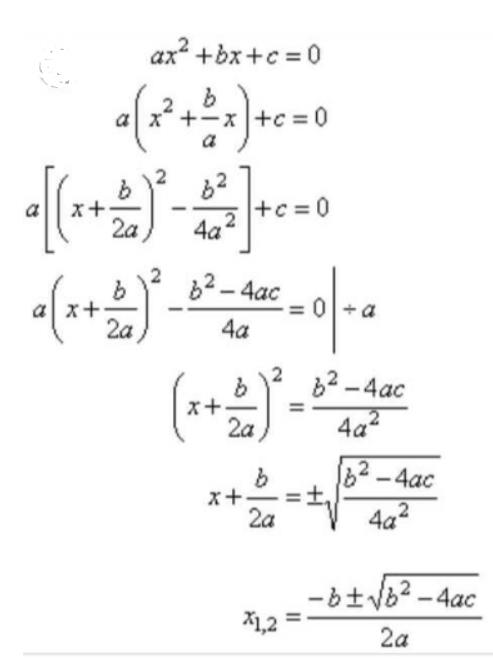Courses

# Test: Method Of Completing The Square

## 10 Questions MCQ Test Mathematics (Maths) Class 10 | Test: Method Of Completing The Square

Description
This mock test of Test: Method Of Completing The Square for Class 10 helps you for every Class 10 entrance exam. This contains 10 Multiple Choice Questions for Class 10 Test: Method Of Completing The Square (mcq) to study with solutions a complete question bank. The solved questions answers in this Test: Method Of Completing The Square quiz give you a good mix of easy questions and tough questions. Class 10 students definitely take this Test: Method Of Completing The Square exercise for a better result in the exam. You can find other Test: Method Of Completing The Square extra questions, long questions & short questions for Class 10 on EduRev as well by searching above.
QUESTION: 1

Solution:
QUESTION: 2

### The equation 4x2 = 4x has following solution/solutions

Solution:

We have 4x2=4x
4x- 4x=0
4x(x-1)=0
Either 4x=0  =  x=0
Or x-1=0  = x=1

QUESTION: 3

### The solution of -9z2 – 4z + 2 = 0 is

Solution:
QUESTION: 4

The solutions of the equationare

Solution:
QUESTION: 5

The roots of quadratic equation are 2x2+3x-9 = 0 are:​

Solution:
QUESTION: 6

The sum of the areas of two squares is 468 m2. If the difference of their perimeters is 24 m, then the sides of the two squares are:​

Solution:
QUESTION: 7

The roots of the quadratic equationare:

Solution:
QUESTION: 8

The difference of a number and its reciprocal is 1.5. Then, the number is/are:

Solution:
QUESTION: 9

The solution of (2x + 1) (x – 5) = 3 is ____

Solution:
QUESTION: 10

The roots of quadratic equation ax2 + bx + c = 0 is given by

Solution: